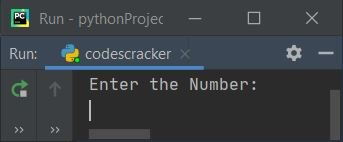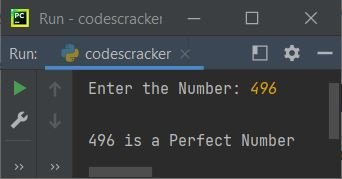# Python Program to Check a Perfect Number

• Check the perfect number using the for loop.
• Check the perfect number using the while loop.

### What is a perfect number?

A perfect number is a number that is equal to the sum of its positive divisors, excluding the number itself. That is, the number 6 has divisors 1, 2, and 3 (excluding 6 itself). And 1+2+3 is equal to 6. Therefore, 6 is a perfect number.

## Check the perfect number using the for loop

The question is: write a program in Python that checks whether a number is perfect or not using a for loop. Here is its answer:

```print("Enter the Number:")
num = int(input())
sum = 0
for i in range(1, num):
if num%i==0:
sum = sum+i
if num==sum:
print("It is a Perfect Number")
else:
print("It is not a Perfect Number")```

Here is its sample run:Now supply the input, say 6, and press the ENTER key to check and print whether it is a perfect number or not, as shown in the snapshot given below:#### Modified version of the previous program

This is the modified version of the previous program, which uses end= and str(). The rest of the program is nearly identical to the previous one. The end= is used to skip the printing of an automatic newline. And str() converts any type into a string type.

```print(end="Enter the Number: ")
num = int(input())
sum = 0
for i in range(1, num):
if num%i==0:
sum = sum+i
if num==sum:
print("\n" + str(num) + " is a Perfect Number")
else:
print("\n" + str(num) + " is not a Perfect Number")```

Here is its sample run with user input (496):## Check the perfect number using the while loop

The question is: write a Python program to check for a perfect number using a while loop. Here is its answer:

```print(end="Enter the Number: ")
num = int(input())
sum = 0
i = 1
while i<num:
if num%i==0:
sum = sum+i
i = i+1
if num==sum:
print("\n" + str(num) + " is a Perfect Number")
else:
print("\n" + str(num) + " is not a Perfect Number")```

Python Online Test

« Previous Program Next Program »